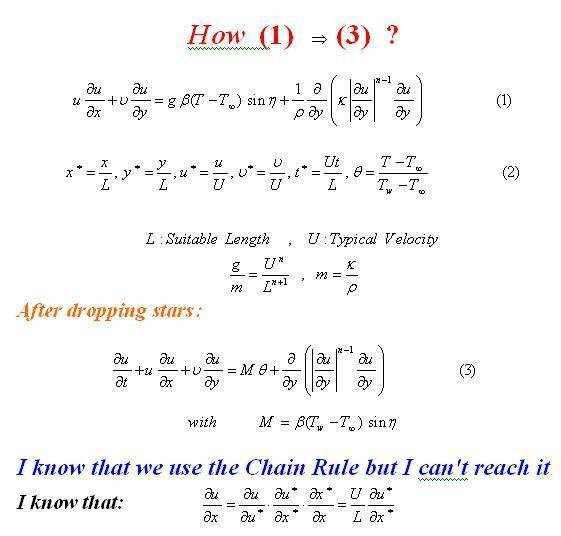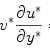# Q about PEDs > I need some help

thepioneerm#### Attachments

coomast
I had a look at it and after a few minutes I got a transformed result, but indeed it is not the one given. However there seems to be a term missing in the left hand side containing the time t. After using the transformation I got (without the term with the time):

$$u^*\frac{\partial u^*}{\partial x^*}+ v^*\frac{\partial u^*}{\partial y^*}= m\cdot \frac{U^{n-1}}{L^n}\cdot \left[M\cdot \theta + \frac{\partial}{\partial y^*} \left[\left|\frac{\partial u^*}{\partial y^*}\right| ^{n-1} \right]\cdot \frac{\partial u^*}{\partial y^*}\right]$$

Maybe the term with t can shed some light on the necessary next step, otherwise I do not see how to reach the end result.

coomast

thepioneerm
coomast

thank you very much

but how do you reach this result ??!!!

please can you explain it to me

even I don't know how to get#### Attachments

coomast
coomast

thank you very much

but how do you reach this result ??!!!

please can you explain it to me

even I don't know how to get

pfff.....the preview gives a different formula then the one I'm typing, I assume the latex is still broken

This is done in the same way as before, you have:

$$v\frac{\partial u}{\partial y}=v^*U\frac{\partial u^*U}{\partial y^*L} = \frac{U^2}{L} v^*\frac{\partial u^*}{\partial y^*}$$

After doing this to the other terms as well, you end up with the result I gave earlier. Beware of the error in the question, you will not be able to reach the solution given.

remark 1: sorry for the late reply, I had a busy week
remark 2: don't use a font this big, it looks as if you are shouting which is not the intention I suppose, instead look at learning the basics of latex, it will be helpfull in producing the nice formula's out here :-) [if the bloody thing works, lol]

seems the latex is OK after all.... this does not follow a mathematical rule, perhaps something chaotic...could be me as well...

best regards,

coomast

thepioneerm
coomast

thank thank thank you very very very much

and I will not use a font this big ^-^

and I will try to learn the basics of latex

thank you